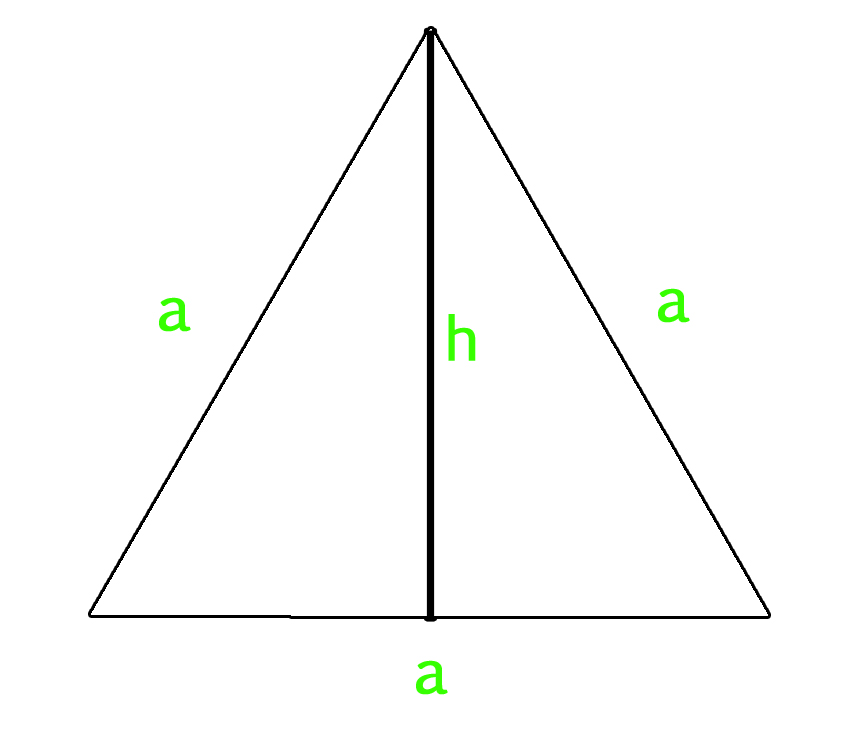# Program to calculate area and perimeter of equilateral triangle

An equilateral triangle is a triangle in which all three sides and angles are equal. All three internal angles of equilateral triangle measures 60 degree.

Area of Equilateral triangle :

• If we know the length of each sides of equilateral triangle, then we can use below mentioned formula to calculate area of equilateral triangle.
`Area of Equilateral Triangle = (sqrt(3)/4) * a * a    `
• If we know the length of altitude of equilateral triangle along with the length of side, then we can use below mentioned formula to calculate it’s area.
`Area of Equilateral Triangle = (1/2) x Side x Altitude`
•
Perimeter of Equilateral Triangle :

`Perimeter of Equilateral Triangle :  3 X a`

How does the area formula work?
Let us take a look at below diagram. We know are of a triangle is 1/2 * base * height. The value of h is sqrt(a2 – (a/2)2) = sqrt(3) * a / 2. So the area becomes 1/2 * a * (sqrt(3) * a / 2) = (sqrt(3)/4) * a * aExamples :

```Input : side = 4
Output : Area of Equilateral Triangle: 6.9282
Perimeter of Equilateral Triangle: 12

Input : side = 12
Output : Area of Equilateral Triangle: 62.3538
Perimeter of Equilateral Triangle: 36
```

## C++

 `// CPP program to find area ` `// and perimeter of equilateral triangle ` `#include ` `using` `namespace` `std; ` ` `  `// Function to calculate Area  ` `// of equilateral triangle ` `float` `area_equi_triangle(``float` `side) ` `{ ` `    ``return` `sqrt``(3) / 4 * side * side; ` `} ` ` `  `// Function to calculate Perimeter  ` `// of equilateral triangle ` `float` `peri_equi_triangle(``float` `side) ` `{ ` `    ``return` `3 * side; ` `} ` ` `  `// Driver Code ` `int` `main() ` `{ ` `    ``float` `side = 4; ` `    ``cout << ``"Area of Equilateral Triangle: "` `         ``<< area_equi_triangle(side) << endl; ` `    ``cout << ``"Perimeter of Equilateral Triangle: "` `         ``<< peri_equi_triangle(side); ` `    ``return` `0; ` `} `

## Java

 `// Java Program to find area and ` `// perimeter of equilateral triangle ` `import` `java.io.*; ` ` `  `class` `GFG  ` `{ ` `    ``// Function to calculate  ` `    ``// Area of equilateral triangle ` `    ``static` `float` `area_equi_triangle(``float` `side) ` `    ``{ ` ` `  `        ``return` `(``float``)(((Math.sqrt(``3``)) / ``4``) * ` `                         ``side * side); ` `    ``} ` ` `  `    ``// Function to calculate  ` `    ``// Perimeter of equilateral ` `    ``// triangle ` `    ``static` `float` `peri_equi_triangle(``float` `side) ` `    ``{ ` `        ``return` `3` `* side; ` `    ``} ` `     `  `    ``// Driver Code ` `    ``public` `static` `void` `main(String arg[]) ` `    ``{ ` `        ``float` `side = ``4``; ` `        ``System.out.print(``"Area of Equilateral Triangle:"``); ` `        ``System.out.println(area_equi_triangle(side)); ` `        ``System.out.print(``"Perimeter of Equilateral Triangle:"``); ` `        ``System.out.println(peri_equi_triangle(side)); ` `    ``} ` `} ` ` `  `// This code is contributed  ` `// by Anant Agarwal. `

## Python

 `# Python3 program to calculate Area and  ` `# Perimeter of equilateral Triangle ` ` `  `# Importing Math library for sqrt ` `from` `math ``import` `*` ` `  `# Function to calculate Area  ` `# of equilateral triangle ` `def` `area_equilateral( side ): ` `    ``area ``=` `(sqrt(``3``) ``/` `4``) ``*` `side ``*` `side ` `    ``print` `(``"Area of Equilateral Triangle: % f"``%` `area) ` ` `  `# Function to calculate Perimeter ` `# of equilateral triangle  ` `def` `perimeter( side ): ` `    ``perimeter ``=` `3` `*` `side ` `    ``print` `(``"Perimeter of Equilateral Triangle: % f"``%` `perimeter) ` `     `  `# Driver code ` `side ``=` `4` `area_equilateral( side ) ` `perimeter( side ) `

## C#

 `// C# Program to find area and ` `// perimeter of equilateral triangle ` `using` `System; ` ` `  `class` `GFG  ` `{ ` `    ``// Function to calculate  ` `    ``// Area of equilateral triangle ` `    ``static` `float` `area_equi_triangle(``float` `side) ` `    ``{ ` ` `  `        ``return` `(``float``)(((Math.Sqrt(3)) / 4) *  ` `                         ``side * side); ` `    ``} ` ` `  `    ``// Function to calculate  ` `    ``// Perimeter of equilateral ` `    ``// triangle ` `    ``static` `float` `peri_equi_triangle(``float` `side) ` `    ``{ ` `        ``return` `3 * side; ` `    ``} ` `     `  `    ``// Driver Code ` `    ``public` `static` `void` `Main() ` `    ``{ ` `        ``float` `side = 4; ` `        ``Console.Write(``"Area of Equilateral Triangle:"``); ` `        ``Console.WriteLine(area_equi_triangle(side)); ` `        ``Console.Write(``"Perimeter of Equilateral Triangle:"``); ` `        ``Console.WriteLine(peri_equi_triangle(side)); ` `    ``} ` `} ` ` `  `// This code is contributed  ` `// by vt_m. `

## PHP

 ` `

Output :

```Area of Equilateral Triangle: 6.9282
Perimeter of Equilateral Triangle: 12```

My Personal Notes arrow_drop_upCheck out this Author's contributed articles.

If you like GeeksforGeeks and would like to contribute, you can also write an article using contribute.geeksforgeeks.org or mail your article to contribute@geeksforgeeks.org. See your article appearing on the GeeksforGeeks main page and help other Geeks.

Please Improve this article if you find anything incorrect by clicking on the "Improve Article" button below.

Improved By : vt_m, nidhi_biet